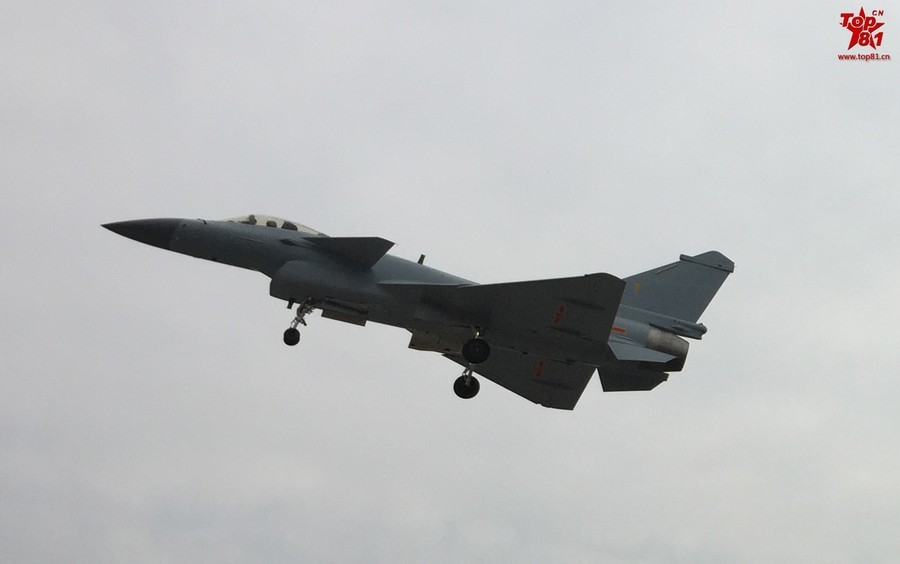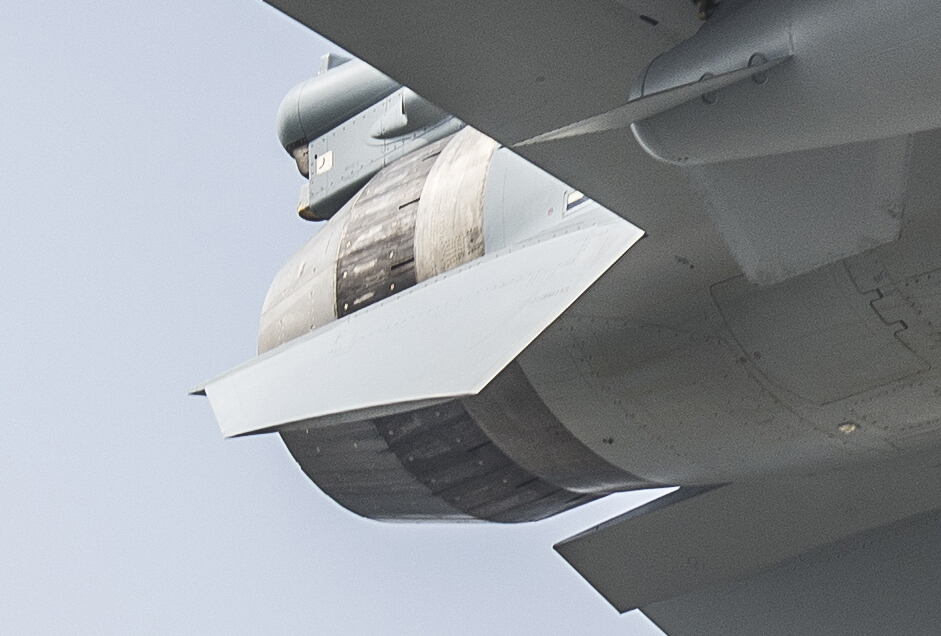Shenyang WS-10 turbofan jet engine

The Shenyang WS-10 (Chinese: 涡扇-10; pinyin: Wōshàn-10; literally: “turbofan-10”), codename Taihang, is a turbofan engine designed and built by the People’s Republic of China.

The WS-10A reportedly powers the J-11B and the J-16. Unconfirmed reports claim the WS-10A powers some J-10Bs. Unconfirmed reports also claim an improved WS-10A powers the J-11D.

Chinese media claimed 266 engines were manufactured from 2010 to 2012 for the J-11 program.Unofficial estimates placed production at more than 300 units by May 2015.

https://en.wikipedia.org/wiki/Shenyang_WS-10

WS-10
WS-10A – improved variant; advertised to have 120–140 kilonewtons (27,000–31,000 lbf) of thrust
WS-10G – thust vectoring variant for the J-20 fighter; 152–155 kilonewtons (34,000–35,000 lbf) of thrust
WS-20 – high-bypass derivative for the Y-20 transport; 138 kilonewtons (31,000 lbf) of thrustKilonewtons

Newton
Unit of Force
Symbol N
Named after Sir Isaac Newton

Newton’s second law of motion states that F = ma, where F is the force applied, m is the mass of the object receiving the force, and a is the acceleration of the object. The newton is therefore:
F = m ⋅ a
1 N = 1 kg ⋅ m/s2

where the following symbols are used for the units:

N: newton
kg: kilogram
m: metre
s: second.

A newton is not much force, so it is common to see forces expressed in kilonewtons, or kN, where 1 kN = 1000 N. For example, the thrust of a an F100 fighter jet is around 130 kN.

Thrust-to-weight ratio: 7.5

The thrust-to-weight ratio can be calculated by dividing the thrust (in SI units – in newtons) by the weight (in newtons) of the engine or vehicle.

Note that the thrust can also be measured in pound-force (lbf) provided the weight is measured in pounds (lb); the division of these two values still gives the numerically correct thrust-to-weight ratio.